# 机器学习基石 Lecture7: The VC Dimension

## Definition of VC Dimension

上节课所讲的N个样本分成的dichotomies数量，也就是假设函数种类的数量，的上限$m_{H}(N)$，小于等于上限函数$B(N,k)$。而$B(N,k)$可以用一个$k-1$维的多项式来限制住。这个$k$叫做Break Point。即：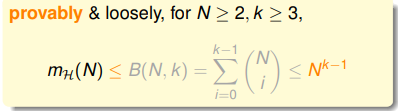因此VC Bound可以进一步的写为：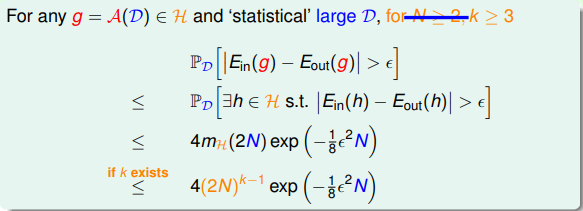因此只要满足三点：

1. $m_{H}(N)$ 有Break Point k （好的假设空间$H$)
2. N 足够大 $\Rightarrow$ probably $E_{out} \approx E_{in}$ （好的数据集$D$
3. 算法$A$选择一个错误率很小的假设函数作为$g$ $\Rightarrow$ probably 能学到东西 （好的算法$A$

对于一个假设空间，$m_{H}(N) = 2^{N}$的最大的$N$叫做这个假设空间的VC 维（VC Dimension）。这个值等于k-1。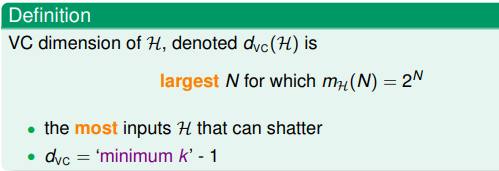下面几个问题的VC维如图：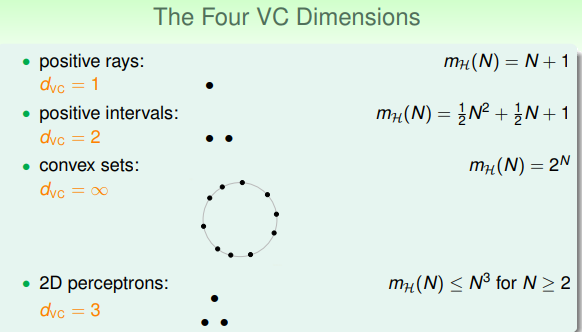因此，如果VC维是有限大的，那么就能够得到一个结果$g$使得$E_{out}(g) \approx E_{in}(g)$。与算法，数据集的采样分布以及目标函数都无关。也就保证了机器学习的泛化性能。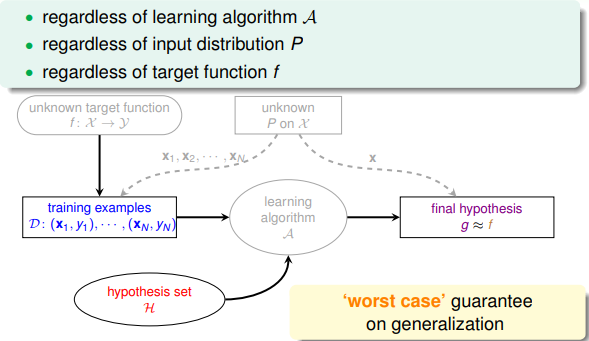## VC Dimension of Perceptrons

回顾一下之前的二位PLA算法。经过了这么多理论推导，我们终于可以相信算法最终能够得到一个比较好的结果。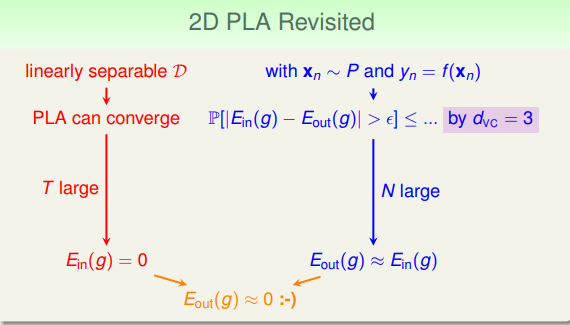对于感知机而言，一维形式的感知机的VC维$d_{vc}=2$，二维感知机VC维$d_{vc}=3$，因此假设$d$维感知机的VC维$d_{vc}=d+1$。为了证明这一点需要证明两个不等式：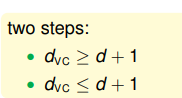首先证明第一个。要证明VC维大于等于d+1，只需要证明存在任意一个数量为d+1的数据集，能够被一个假设函数分成$2^{d+1}$种形式即可。因此我们来设定一个特殊的输入，每个样本$x_{i}$的第0位和第i-1位为1，组成输入矩阵如下：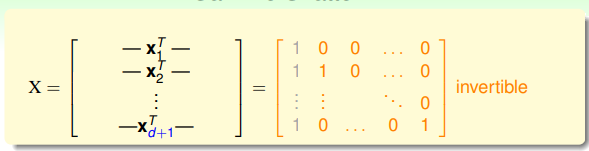这个矩阵是可逆的。因此对于任意的输出向量$y$，对应的系数$w$都是有解的。而y每一项只有+1和-1两种选择，因此存在$2^{d+1}$种对应的y，也就是存在$2^{d+1}$种对应的w，即假设函数h。于是得证。

下面证明第二个不等式。需要证明的是对于任意数量为d+2的数据集，都不能够将对应的结果分类为$2^{d+2}$种形式。先看一个二维形式下对应的4个数据点的例子：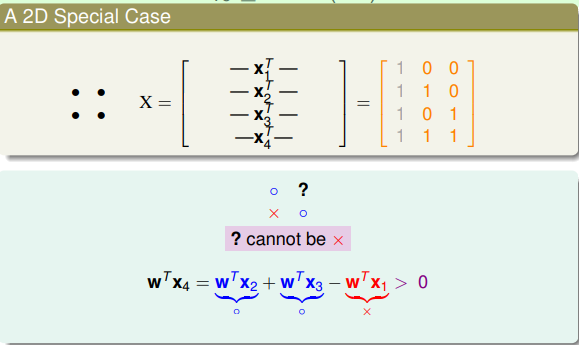假设左上右下两个为正，左下为负。图中的例子里不同的$x_{i}$有一个等式。这个等式决定了$x_{4}$对应的点不可能被分为-1。因此大致可以看出$d+2$时不同样本$x_{i}$的线性相关性导致了不能得到$2^{d+2}$个分类方式。更一般的，对于d维形式的感知机，每个数据点$x_{i}$的维度为$d+1$(第一位均为1），因此我们有一个长$d+2$$d+1$的矩阵。根据线性代数的知识，这d+2个向量必然是线性相关的，即$x_{d+2}$可以写为其它的$x_{i}$的线性组合的形式。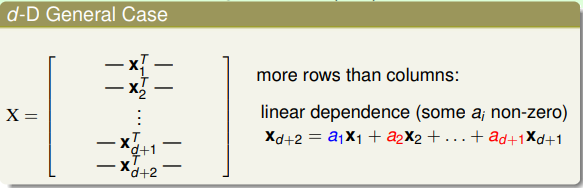如果$x_{1}...x_{d+1}$对应的结果与其系数$a_{i}$的符号相同，这时$x_{d+2}$的结果必定是+1，也就是说必定缺少了一种结果的分配方式。因此得证。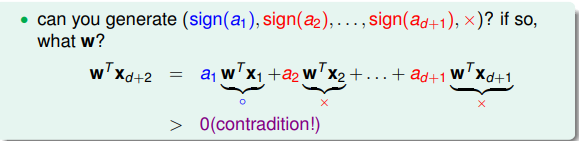两个不等式都证明完毕，因此d维感知机的VC维$d_{vc}=d+1$

## Physical Intuition of VC Dimension

那么VC维代表的是什么物理意义呢？对于每一个假设函数而言我们都有一个不同维度的系数$w$，改变这个系数向量中的每一维就表示对这个假设函数进行调整。因此这个系数向量表示了假设函数的自由度。而假设空间的数量$M=|H|$类似的表达了这个自由度的概念。而VC维就相当于这些自由度的维度，在多少个有效的维度上改变这些自由度才是有用的。而自由度的维度往往也表示了一个假设函数的拟合目标函数的能力大小。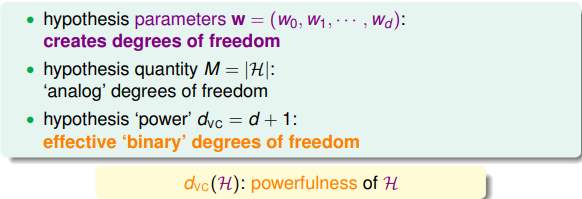举个之前的例子：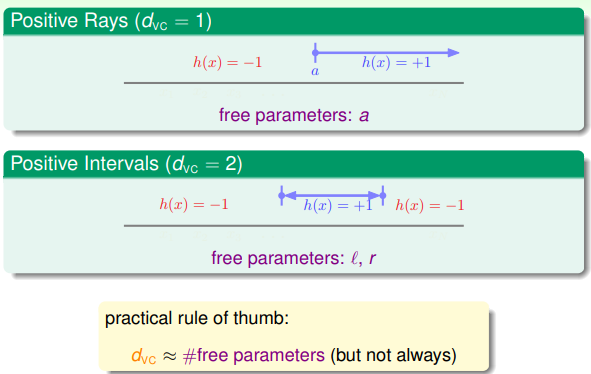很多时候VC维可以近似看成自由系数的个数，当然不完全是。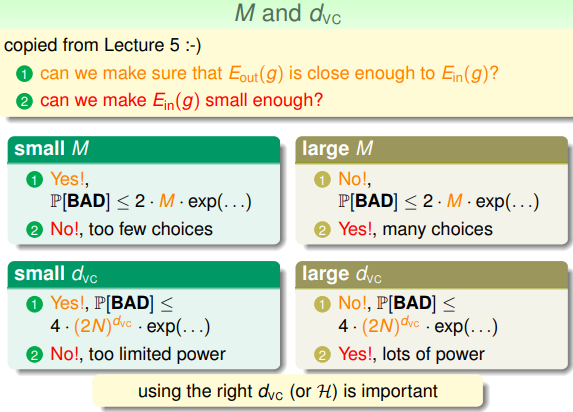## Interpreting VC Dimension

VC维可以看成是一种对于模型复杂度的描述。而且在实际应用中会因为复杂度导致一定的penalty。在不等式中可以看到VC维的作用：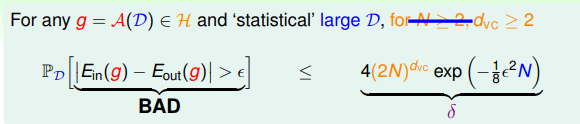当固定了内部错误率和外部错误率之间的差别$\epsilon$时我们可以看出，越大的VC维就会导致越大的概率上限，有可能会导致这个概率上限大于1而没有了意义，也就导致了内部错误率和外部错误率无法再近似相等。

而当我们固定了概率为$\delta$时，对应的差别$\epsilon$可以写为VC维的函数：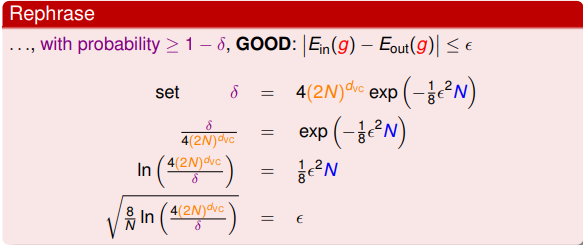因此内部错误率和外部错误率的差别是VC维的函数，当VC维很大时也会导致它们之间的差别过大不等式无法再起效果。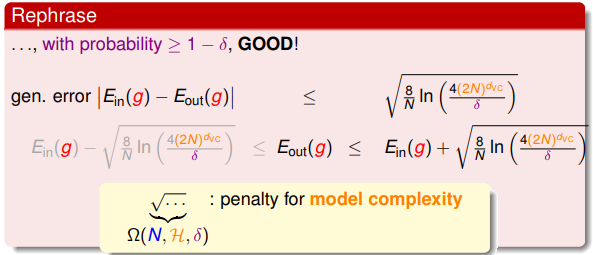通常来说，当其它变量保持不变时，VC维与内部错误率，外部错误率的关系如下图所示：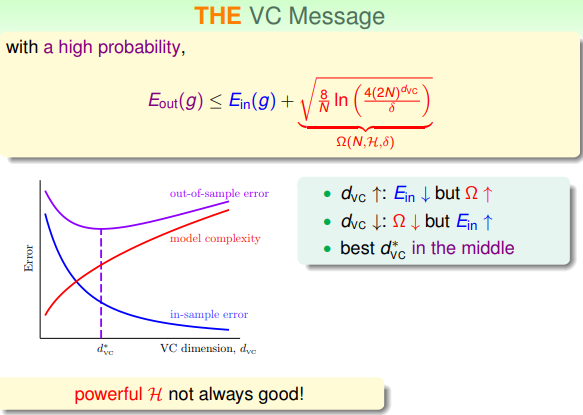那么我们究竟需要多少个样本才能够保证得到一个比较可信的结果呢？根据上限的公式算出来理论的数量是$N\approx 10000d_{vc}$，但实际使用中$N\approx 10d_{vc}$就可以得到不错的效果。实际上这是由于VC维以及在不等式的推导中使用了非常多的放缩，使得整个不等式的上限对于实际情况非常的宽松。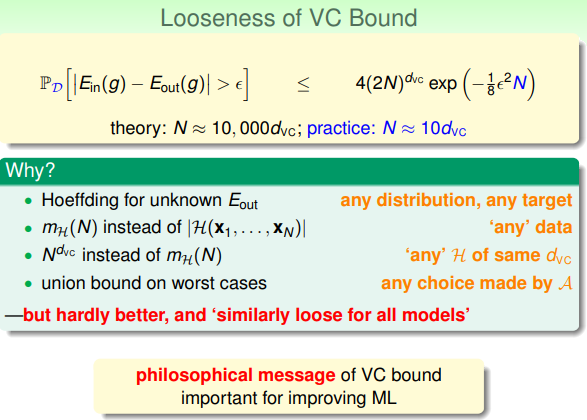但是虽然宽松，但是基本上对于所有的模型，这些宽松的程度是很接近的，也就是说不同模型的VC维以及不等式的使用是一个可比较的项。而且也找不到其他的量能够像VC维一样有力地促进模型和算法的改进。

还能输入1000个字符没有更多推荐了，返回首页